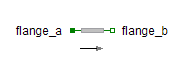Add - MapleSim Help

# Online Help

###### All Products    Maple    MapleSim

Home : Support : Online Help : MapleSim : MapleSim Component Library : Signal Blocks : Mathematical : Operators : componentLibrary/signalBlocks/math/operators/Add

Add

Add the inputsDescription The Add component computes the element-wise addition of the inputs. The Signal Size parameter assigns the dimension of the input and output connectors.
 Equations ${y}_{j}={k}_{1}{\mathrm{u1}}_{j}+{k}_{2}{\mathrm{u2}}_{j}$

Connections

 Name Description Modelica ID $\mathrm{u1}$ Real input vector u1 $\mathrm{u2}$ Real input vector u2 $y$ Real output vector y

Parameters

 Name Default Units Description Modelica ID ${k}_{1}$ $1$ Gain of upper input k1 ${k}_{2}$ $1$ Gain of lower input k2 Signal Size $1$ Dimension of input and output signals signalSize

 Modelica Standard Library The component described in this topic is from the Modelica Standard Library. To view the original documentation, which includes author and copyright information, click here.

 See Also